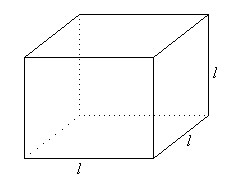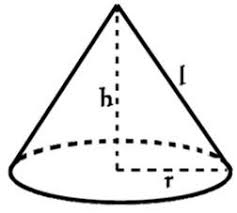We know there are many other things you would rather do than learn how to calculate the surface area of various geometric shapes. You could go to a party, hang out with friends, watch a movie, binge-watch the series on Netflix that everyone won’t shut up about, do laundry, clean your room. Okay, maybe we’re getting ahead of ourselves here. But there are perks to knowing how to calculate the surface area of objects. For one, if you’re a sucker for chocolate cake, you’ll need to know the surface area of the cake in order use the right amount of frosting. Ever wonder why the new pair of heels you bought kills your feet after walking for two minutes? Answer: Surface area. Area calculations find their way into our daily lives in one way or another. Hence, knowing how to calculate the surface area of basic objects is important. Let’s break down the basics of what surface area is and figure out how to calculate the surface area for various geometric objects.Image Credits: Rohrlack-Chute Middle School

The surface area of an object includes the sum of all the areas of the faces that cover the object. Generally, “surface area” is used in relation to three-dimensional objects which have various faces, and may be comprised of different shapes.

## Some Major Objects and their Surface Area Formulas

 SHAPES FORMULA DESCRIPTION Rectangular Prism2ab + 2bc + 2ac The length of the three sides are marked with a, b, and c. The area of each surface can be obtained from multiplying the length and height or the length and width. Since there are two of each surface, each of these areas is multiplied by 2. Cube6a2 Since a cube is comprised of six square surfaces, and the area of each square is derived by multiplying the length and width (a X a = a2), the area of the square is multiplied by 6. Sphere4πr2 A long time ago, an old man named Archimedes published two volumes of work entitled On the Sphere and Cylinder. In it, he uses mathematical formulas to explain that the surface area of a sphere is four times the area of its great circle, a circle that divides a sphere in two equal hemispheres.   Hence, the radius of the circle is squared (r2), multiplied by π, and the product is multiplied by 4. Cylinder2πr2 + 2πrh There are two different shapes that make up the surfaces of a cylinder. The top and the base are made of circles, and the body, if unwrapped is the shape of a rectangle.   To get the area of the circles, we use πr2, which is multiplied by 2 because there are two circles. The surface area of the rectangle requires multiplying the length and height twice. In this case, the length can be ascertained by the circumference of the 2πr. To get the surface area of the rectangle, the circumference of the circle (2πr) is multiplied by the height. Each of these components is then added to get the total surface area of a cylinder. Square Pyramids2 + 2s(l) There are two shapes that make up the square pyramid: 4 triangles and a square base. The first part of the formula (s2)* gives us the area of the square base. The second part of the formula requires a further breakdown. As you’ll notice, the four triangles are slanted. In order to find the surface area, we need to account for this slant. As we know, the formula for the area of a triangle is ½ s(l); since there are four triangles, we multiply that formula by four to get: 4 x ½ s(l) = 2 (s)(l). Hence, the total surface area can be derived by adding the two components together: s2 + 2s(l). *Note: s is used to denote the value of a side, and l is used to denote the value of the slant height. Coneπr2 + πrl There are two components to a cone: a circular base, and a triangular body, with a slant and a curved edge. The first part of the formula accounts for the area of a circle (πr2).  The second part multiplies the slant height, l, with the radius and pi, in order to get the surface area of the body of the cone. Both components of the formula are summed to get the total surface area of the cone.

Thus, this lesson provides a cursory overview of how to calculate the surface area of some key geometric shapes and breaks down each formula to make it easier to grasp the fundamental concept behind its calculation. Using the knowledge from this article, you can figure out the surface area of other, more complex shapes. Next time, when a pair of shoes feel uncomfortable, you’ll know that the amount of surface area is inadequate for your feet to distribute the weight of your body evenly, making it difficult to walk and look cool at the same time.

To read more knowledge articles on geometric shapes, visit here!

## Shock your Mom with more marks than she expected

Get access to 300,000+ questions curated by India’s best academic experts.

+91
No thanks.

## Request a Free 60 minute counselling session at your home

Please enter a valid phone number
•7,829,648

Happy Students
•358,177,393

Questions Attempted
•3,028,498

Tests Taken
•3,020,367